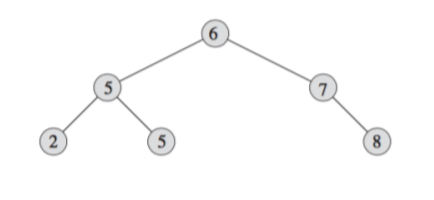# Binary search tree using JavaScript ES6-Typescript

0

A binary search tree is a data structure composed of nodes. Each node has a key, which determines the node’s position in the tree. (The node may also have a “value” field, where additional data is stored.) … Specifically, each node has a left child, a right child, and a parent (some of which may be NIL).In this post, I will show you how to create a binary search tree javascript es6/typescript.``````class TreeNode {
constructor(
public data: number,
public left: TreeNode = null,
public right: TreeNode = null
) {}
}

``````
``````export class BinarySearchTree {
constructor(private root: TreeNode = null) {}

public insert(data: number): void {
let newNode = new TreeNode(data);
if (!this.root) {
this.root = newNode;
return;
}

let current = this.root;
while (true) {
if (data < this.root.data) {
if (current.left == null) {
current.left = newNode;
return;
}
current = current.left;
} else if (data > this.root.data) {
if (current.right == null) {
current.right = newNode;
return;
}
current = current.right;
}
}
}

}
``````
Tags